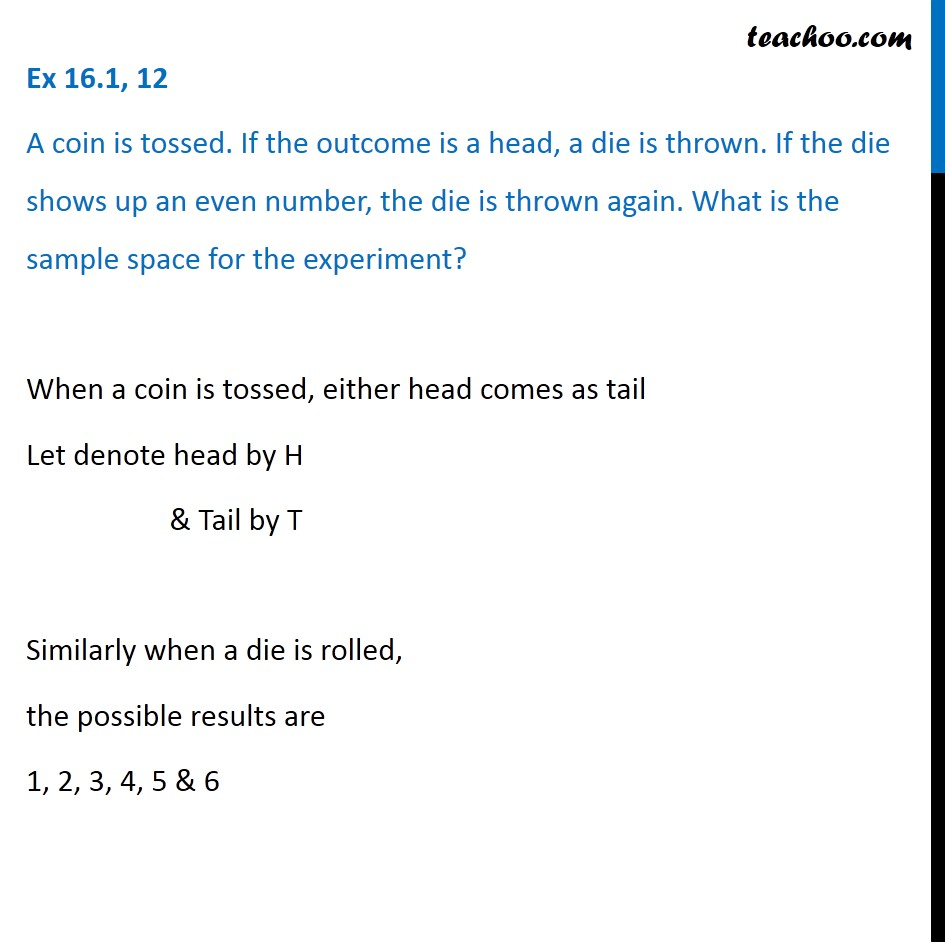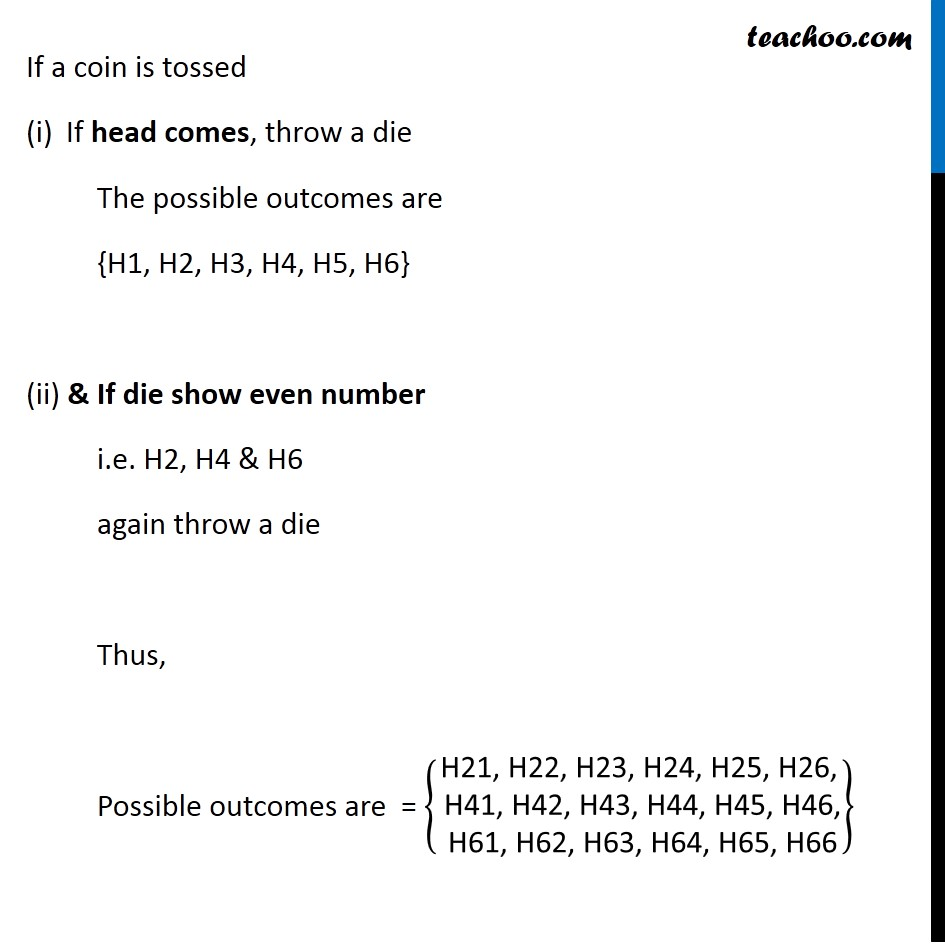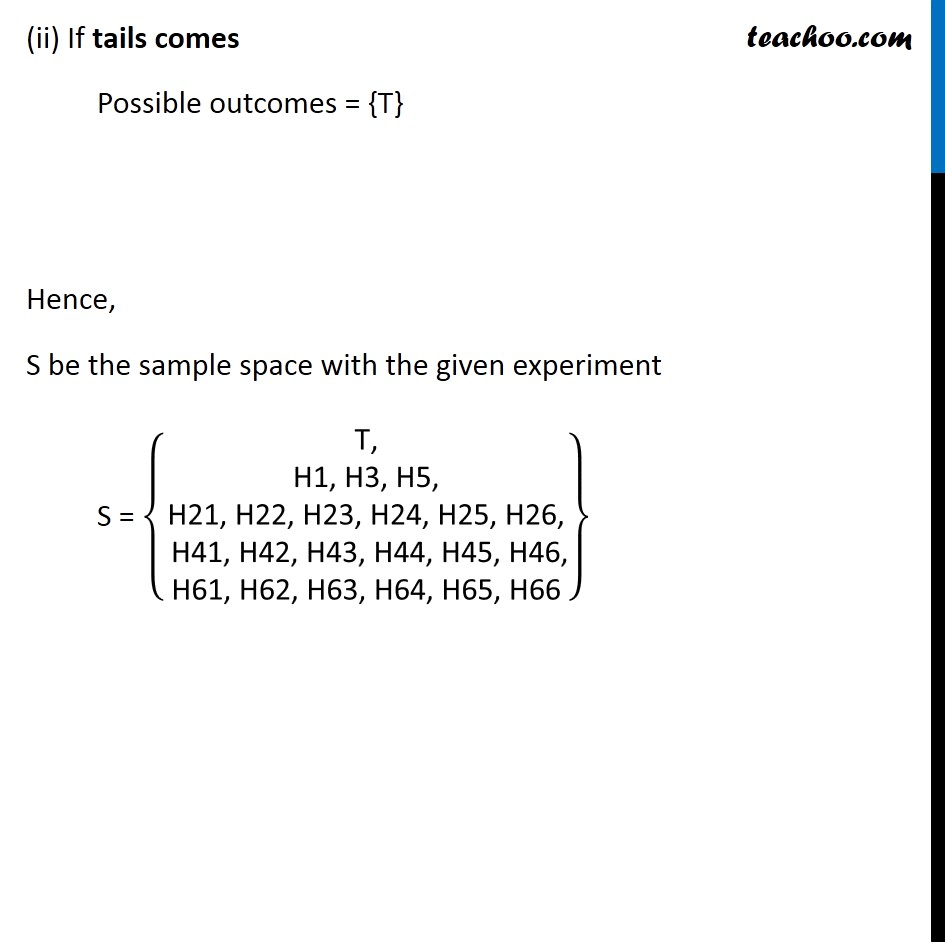1. Chapter 16 Class 11 Probability
2. Concept wise
3. Sample Space

Transcript

Ex 16.1, 12 A coin is tossed. If the outcome is a head, a die is thrown. If the die shows up an even number, the die is thrown again. What is the sample space for the experiment? When a coin is tossed, either head comes as tail Let denote head by H & Tail by T Similarly when a die is rolled, the possible results are 1, 2, 3, 4, 5 & 6 If a coin is tossed If head comes, throw a die The possible outcomes are {H1, H2, H3, H4, H5, H6} (ii) & If die show even number i.e. H2, H4 & H6 again throw a die Thus, Possible outcomes are = {█("H21, H22, H23, H24, H25, H26," @" H41, H42, H43, H44, H45, H46," @" H61, H62, H63, H64, H65, H66" )} (ii) If tails comes Possible outcomes = {T} Hence, S be the sample space with the given experiment S = {█("T," @" H1, H3, H5, " @"H21, H22, H23, H24, H25, H26," @" H41, H42, H43, H44, H45, H46," @"H61, H62, H63, H64, H65, H66" )}

Sample Space